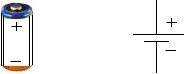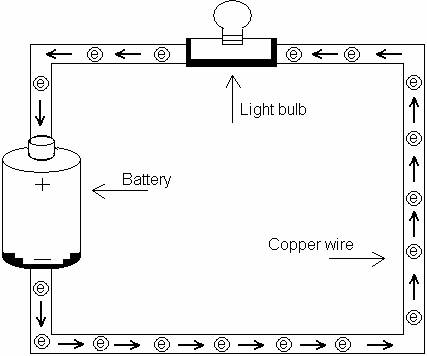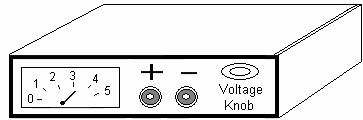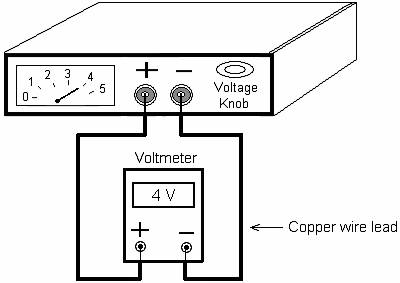DC Voltage and Current:  An example of a dc voltage source is a

battery. Figure 1.1 shows a battery and its schematic diagram.Figure 1.1

Figure 1.2 shows the battery forces the free electrons (particles with negative charge) in the copper wire to flow from the negative pole of the battery toward the positive pole of the battery (flow of electrons is in one direction). Note that the light bulb is ‘ON’ due to flow of electrons in this closed loop.

The voltage of a battery is measured in volts (V).Figure 1.2

Note: The diagram in figure 1.2 is a closed loop. This closed loop is called a circuit.

The flow of electron in the circuit of figure 1.2 is similar to the water-pump which forces the flow of water in a pipe or hose in one direction. Therefore water-pump acts as battery and the flow of water is similar to the flow of electrons.

The flow of the electrons in the wire is called Electric Current. The electric current is

measured in Amperes (Amps).

Power Supply: Figure 1.5 shows a typical power supply.Figure 1.5

In figure 1.5, this power supply provides dc voltage and current similar to a regular battery. A power supply is a device that converts AC voltage from the wall outlet into DC voltage.

The main application of a power supply is its capability of supplying varying dc voltage and current to an electronic component.

Note that the schematic symbol of a battery can be used to represent a power supply in a circuit.

Voltmeter: In order to measure the voltage across a voltage source or an

electrical component, a Voltmeter is used. Figure 1.6 shows a voltmeter connected with a power supply.Figure 1.6

Figure 1.7 shows a voltmeter connected with a 1.5 volts battery.Figure 1.7